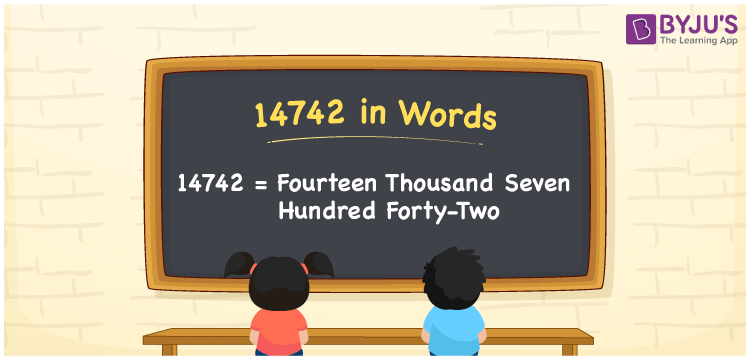# 14742 in Words

14742 in words is written as Fourteen thousand seven hundred forty-two. In both the International System of Numerals and the Indian System of Numerals, 14742 is written as Fourteen thousand seven hundred forty-two. The number 14742 is a Cardinal Number as it denotes some quantity. For example, “that mobile phone costs 14742 rupees”.

 14742 in Words Fourteen thousand seven hundred forty-two Fourteen thousand seven hundred forty-two in Number 14742

## 14742 in English Words

14742 in English words is read as “Fourteen thousand seven hundred forty-two”.## How to Write 14742 in Words?

To write 14742 in words, we shall use the place value chart. In the place value chart, put 1 in the ten thousands, 4 in the thousands, 7 in the hundreds, 4 in the tens and 2 in the ones. Let us make a place value chart to write the number 14742 in words.

 Ten Thousands Thousands Hundreds Tens Ones 1 4 7 4 2

Thus, we can write the expanded form as

1 × Ten Thousand + 4 × Thousand + 7 × Hundred + 4 × Ten + 2 × One

= 1 × 10000 + 4 × 1000 + 7 × 100 + 4 × 10 + 2 × 1

= 10000 + 4000 + 700 + 40 + 2

= 14742

= Fourteen thousand seven hundred forty-two.

14742 is a natural number, the successor of 14744 and the predecessor of 14746.

14742 in words – Fourteen thousand seven hundred forty-two

• Is 14742 an odd number? – No
• Is 14742 an even number? – Yes
• Is 14742 a perfect square number? – No
• Is 14742 a perfect cube number? – No
• Is 14742 a prime number? – No
• Is 14742 a composite number? – Yes

## Frequently Asked Questions on 14742 in Words

Q1

### How to write 14742 in words?

14742 in words is written as Fourteen thousand seven hundred forty-two.
Q2

### How to write 14742 in the International and Indian System of Numerals?

In both, the system of numerals, 14742 in words, is written as Fourteen thousand seven hundred forty-two.
Q3

### How to write 14742 in a place value chart?

In the place value chart, write 1 in the ten thousands, 4 in the thousands, 7 in the hundreds, 4 in the tens and 2 in the ones, respectively.